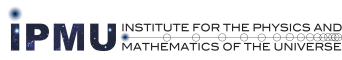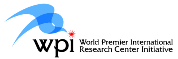### A Workshop on Micro-local analysis on symplectic manifolds

In mathematics, functions on a manifold form a commutative algebra, however in quantum physics, they are not commutative but the differences of right and left products are power seires of $\hbar$. Such phenomenon is called deformation quantization.
In the present workshop, we shall have a basic course by Masaki Kashiwara on the category of sheaves of deformation quatization on a symplectic manifold with the Frobenius action and its application to representation theory of rational Cherednik algebras. We shall have related lectures by Berard Leclerc, Toshiro Kuwabara and Kentaro Nagao.

Date: September 16 - 18, 2008
Place: Seminar Room at IPMU Prefab. B, Kashiwa Campus of the University of Tokyo
Organizer: Kyoji Saito(IPMU)
Speaker:
• Masaki Kashiwara (KURIMS, IPMU)
• Toshiro Kuwabara (KURIMS)
• Bernard Leclerc (Universite de Caen)
• Kentaro Nagao (Kyoto)
Schedule:
• 16 (Tuesday)
 10:30 - 11:30 Kashiwara lunch 13:30 - 14:30 Leclerc break 15:00 - 16:00 Kuwabara

• 17 (Wednesday)
 10:30 - 11:30 Kashiwara lunch 13:30 - 14:30 Leclerc break 15:00 - 16:00 Nagao

• 18 (Thursday)
 10:30 - 11:30 Kashiwara lunch 13:30 - 14:30 Leclerc break

Title and abstract:
Masaki Kashiwara (KURIMS, IPMU)

"Quantization of symplectic manifolds"

The deformation quantization of a symplectic manifold is a sheaf of rings on this symplectic manifold. It contains a parameter $\hbar$, so that the category of modules over this ring is a $\mathbd{C}[[\hbar]]$-linear category. In the application, we need to eliminate $\hbar$ and to obtain $\mathbf{C}$-linear category. In order to perform this, we propose the notion of Frobenius action. I also explain its application to the representation theory of rational Cherednik algebras.

Toshiro Kuwabara (KURIMS)

"Characteristic cycles of standard modules for the rational Cherednik algebra of type Z / l Z"

We study the representation theory of the rational Cherednik algebra $H_\kappa = H_\kappa({\mathbb Z}_l)$ for the cyclic group ${\mathbb Z}_l = {\mathbb Z} / l {\mathbb Z}$ and its connection with the geometry of the quiver variety $\mathfrak{M}_\theta(\delta)$ of type $A_{l-1}^{(1)}$.

We consider a functor between the categories of $H_\kappa$-modules with different parameters, called the shift functor, and give the condition when it is an equivalence of categories.

We also consider a functor from the category of $H_\kappa$-modules with good filtration to the category of coherent sheaves on $\mathfrak{M}_\theta(\delta)$. We prove that the image of the regular representation of $H_\kappa$ by this functor is the tautological bundle on $\mathfrak{M}_\theta(\delta)$. As a corollary, we determine the characteristic cycles of the standard modules. It gives an affirmative answer to a conjecture given in \cite{Go} in the case of ${\mathbb Z}_l$.

Bernard Leclerc (Universite de Caen)

"Nilpotent varieties and cluster algebras"

1. Lusztig's lagrangian construction of the enveloping algebra U(n) of the positive part n of a Kac-Moody algebra g. This involves constructible functions on the so-called nilpotent varieties. This yields a basis of U(n) called the semicanonical basis. It is an open problem how this basis compares with the canonical (or global) basis of the quantum algebra U_q(n) specialized at q=1.
2. For simplicity, assume that g is finite-dimensional. The graded dual of U(n) is isomorphic to the coordinate ring C[N] where N is the unipotent group with Lie(N) = n. Thus C[N] has a dual semicanonical (and a dual canonical basis). Fomin and Zelevinsky have introduced a cluster algebra structure on C[N]. (I will recall what this means). One of our main results is that the cluster monomials of C[N] belong to the dual semicanonical basis. They are naturally labelled by the irreducible components of the nilpotent varieties which have an open orbit under the group of base change transformations. The main idea is to remember that Lusztig's nilpotent varieties are representation varieties of the Gelfand-Ponomarev preprojective algebra. So we can use some machinery of the representation theory of finite-dimensional algebras.
3. Generalization of (2) to the following cases:
• (a) N is replaced by the unipotent radical of a standard parabolic subgroup of G.
• (b) N is replaced by the unipotent subgroup N(w) = N \cap w^{-1} N_{-} w where w belongs to the Weyl group and N_{-} is the opposite maximal unipotent.
In fact (b) makes sense in the Kac-Moody case because N(w) is always finite-dimensional.

Kentaro Nagao (Kyoto)

"Flop invariance of curve counting on Calabi-Yau 3-folds"

I will prove the flop invariance of euler numbers of Donaldson-Thomas and Pandharipande-Thomas moduli spaces on Calabi-Yau $3$-folds. These moduli spaces can be realized as moduli spaces of "perverse coherent systems" at specific stability parameters. The flop invariance follows as an application of Joyce's wall-crossing formula.